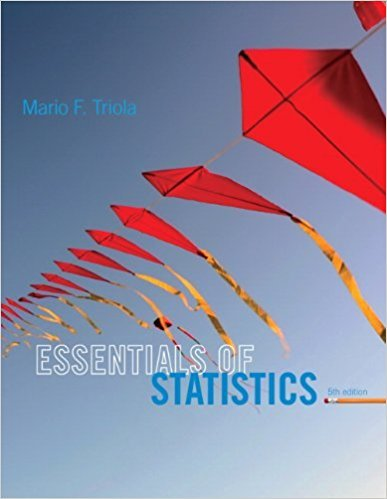×
×

# Solutions for Chapter 5.4: Parameters for Binomial Distributions## Full solutions for Essentials of Statistics | 5th Edition

ISBN: 9780321924599Solutions for Chapter 5.4: Parameters for Binomial Distributions

Solutions for Chapter 5.4
4 5 0 363 Reviews
11
3
##### ISBN: 9780321924599

This expansive textbook survival guide covers the following chapters and their solutions. This textbook survival guide was created for the textbook: Essentials of Statistics, edition: 5. Chapter 5.4: Parameters for Binomial Distributions includes 23 full step-by-step solutions. Essentials of Statistics was written by and is associated to the ISBN: 9780321924599. Since 23 problems in chapter 5.4: Parameters for Binomial Distributions have been answered, more than 14858 students have viewed full step-by-step solutions from this chapter.

Key Statistics Terms and definitions covered in this textbook
• 2 k p - factorial experiment

A fractional factorial experiment with k factors tested in a 2 ? p fraction with all factors tested at only two levels (settings) each

• Additivity property of x 2

If two independent random variables X1 and X2 are distributed as chi-square with v1 and v2 degrees of freedom, respectively, Y = + X X 1 2 is a chi-square random variable with u = + v v 1 2 degrees of freedom. This generalizes to any number of independent chi-square random variables.

• Binomial random variable

A discrete random variable that equals the number of successes in a ixed number of Bernoulli trials.

• Central composite design (CCD)

A second-order response surface design in k variables consisting of a two-level factorial, 2k axial runs, and one or more center points. The two-level factorial portion of a CCD can be a fractional factorial design when k is large. The CCD is the most widely used design for itting a second-order model.

• Comparative experiment

An experiment in which the treatments (experimental conditions) that are to be studied are included in the experiment. The data from the experiment are used to evaluate the treatments.

• Conidence interval

If it is possible to write a probability statement of the form PL U ( ) ? ? ? ? = ?1 where L and U are functions of only the sample data and ? is a parameter, then the interval between L and U is called a conidence interval (or a 100 1( )% ? ? conidence interval). The interpretation is that a statement that the parameter ? lies in this interval will be true 100 1( )% ? ? of the times that such a statement is made

• Contour plot

A two-dimensional graphic used for a bivariate probability density function that displays curves for which the probability density function is constant.

• Convolution

A method to derive the probability density function of the sum of two independent random variables from an integral (or sum) of probability density (or mass) functions.

• Correction factor

A term used for the quantity ( / )( ) 1 1 2 n xi i n ? = that is subtracted from xi i n 2 ? =1 to give the corrected sum of squares deined as (/ ) ( ) 1 1 2 n xx i x i n ? = i ? . The correction factor can also be written as nx 2 .

• Crossed factors

Another name for factors that are arranged in a factorial experiment.

• Deming

W. Edwards Deming (1900–1993) was a leader in the use of statistical quality control.

• Deming’s 14 points.

A management philosophy promoted by W. Edwards Deming that emphasizes the importance of change and quality

• Discrete distribution

A probability distribution for a discrete random variable

• Discrete uniform random variable

A discrete random variable with a inite range and constant probability mass function.

• Erlang random variable

A continuous random variable that is the sum of a ixed number of independent, exponential random variables.

• Experiment

A series of tests in which changes are made to the system under study

• False alarm

A signal from a control chart when no assignable causes are present

• Fraction defective control chart

See P chart

• Gamma random variable

A random variable that generalizes an Erlang random variable to noninteger values of the parameter r

• Generator

Effects in a fractional factorial experiment that are used to construct the experimental tests used in the experiment. The generators also deine the aliases.

×